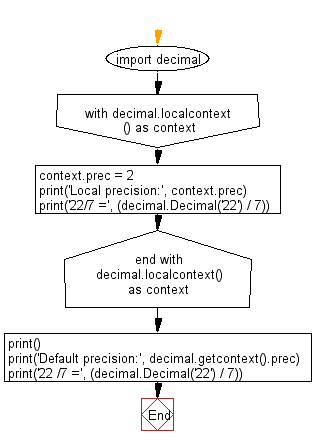﻿ Python Math: Get the local and default precision - w3resource# Python Math: Get the local and default precision

## Python Math: Exercise-42 with Solution

Write a Python program to get the local and default precision.

Sample Solution:-

Python Code:

``````import decimal
with decimal.localcontext() as context:
context.prec = 2
print('Local precision:', context.prec)
print('22/7 =', (decimal.Decimal('22') / 7))

print()
print('Default precision:', decimal.getcontext().prec)
print('22 /7 =', (decimal.Decimal('22') / 7))
```
```

Sample Output:

```Local precision: 2
22/7 = 3.1

Default precision: 28
22 /7 = 3.142857142857142857142857143
```

Flowchart:Python Code Editor:

Have another way to solve this solution? Contribute your code (and comments) through Disqus.

What is the difficulty level of this exercise?

Test your Programming skills with w3resource's quiz.

﻿

```>>> students = [{'name': 'John', 'score': 98}, {'name': 'Mike', 'score': 94}, {'name': 'Jennifer', 'score': 99}]